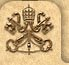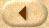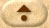Help
 Alphabetical    [«  »]introduced 45introduces 16introducing 5introduction 144introductions 8introductory 8intrude 1 Frequency    [«  »]145 religious145 speaks145 vision144 introduction144 slain143 ancestors143 anointed New American Bible 2002 11 11IntraText - Concordancesintroduction
```                                                                     bold = Main text
Part, Book  Chapter:Verse                                        grey = Comment text
1  PreNAB                     |            new features: a general introduction to the Pentateuch, a retranslation
2  PreNAB                     |            text of Genesis with an introduction, cross-references, and revised
3  PreNAB                     |            narrative. And with the introduction of Abraham and his covenant
4  PreNAB                     |         older oral traditions (see Introduction to the Pentateuch).~ ~In
5    Pent,  Gen   26:   34(6) |     preceding stories, serve as an introduction to the following section
6    Pent,  Exo               |                             Exodus~Introduction ~The second book of the
7    Pent,  Lev  Int          |                                    Introduction~The name "Leviticus" was
8    Pent,  Num  Int          |                                    Introduction ~The Book of Numbers derives
9    Pent,  Deu  Int          |                                    Introduction~The fifth and last book
10    Pent,  Deu   33:    2(1) |          33:2-5) serves here as an introduction to the various "blessings";
11    Pent,  Jos  Int          |                                    Introduction ~The Book of Joshua derives
12    Pent,  Jud  Int          |                                    Introduction ~The Book of Judges derives
13    Pent,  Rut  Int          |                                    Introduction ~The Book of Ruth is named
14    Pent,  Rut  Int          |           which it is an excellent introduction, since it traces the ancestry
15     His, 1Sam   11:    1(1) |      ancient evidence for a longer introduction to this campaign. The time
16     His, 1Kin  Int          |                                    Introduction~The two Books of Kings were
17     His, 2Kin   16:   15(2) |          consultation: perhaps the introduction into Judah of the Babylonian
18     His, 1Chr  Int          |                                    Introduction~Originally the two books
19     His, 2Chr  Int          |                                    Introduction~The Second Book of Chronicles
20     His,  Ezr  Int          |                                    Introduction~The last four books of the
21     His,  Neh  Int          |                                    Introduction~Problems common to the combined
22     His,  Neh  Int          |            been pointed out in the Introduction to the Book of Ezra. The
23     His,  Neh   13:    1(1) |          Chronicler to serve as an introduction to the reforms that Nehemiah
24     His,  Tob  Int          |                                    Introduction~The Book of Tobit, named
25     His,  Tob    1:   15(6) |          is a religious novel (see Introduction; also notes on Tobit 5:6;
26     His,  Tob    4:    3(1) |    especially Prov and Sirach (see Introduction): duties toward parents (
27     His,  Tob    5:    6(3) |       notes on Tobit 1:15; 3:7 and Introduction.)~
28     His,  Jdt  Int          |                                    Introduction~The Book of Judith is a
29     His,  Est  Int          |                                    Introduction~The Book of Esther is named
30     His, 1Mac  Int          |                                    Introduction~The name Maccabee, probably
31     His, 1Mac  Int          |                       I.           Introduction: Hellenism in Asia Minor (
32     His, 2Mac  Int          |                                    Introduction~Although this book, like
33   WisdB,  Job  Int          |                                    Introduction~The Book of Job, named after
34   WisdB,  Psa  Int          |                                    Introduction~The Hebrew Psalter numbers
35   WisdB,  Psa   18:    1(1) |          report has a short hymnic introduction (Psalm 18:2-4, 32-36) and
36   WisdB,  Psa   18:    8(5) |           comes from the west. The introduction to the theophany (Psalm
37   WisdB,  Psa   78:    1(1) |           of David? The tripartite introduction invites Israel to learn
38   WisdB,  Pro  Int          |                                    Introduction~The first word of this book,
39   WisdB,  Pro  Int          |                       I.           Introduction: The Value of Wisdom (Proverb
40   WisdB,  Ecc  Int          |                                    Introduction~The title Ecclesiastes given
41   WisdB,  Son  Int          |                                    Introduction~The Song of Songs, meaning
42   WisdB, Wisd  Int          |                                    Introduction~The Book of Wisdom was written
43   WisdB,  Sir  Int          |                                    Introduction~The Book of Sirach derives
44   WisdB,  Sir   18:   28(5) |         preceding section or as an introduction to the following one. The
45  ProphB,  Isa  Int          |                                    Introduction~The greatest of the prophets
46  ProphB,  Jer  Int          |                                    Introduction~The Book of Jeremiah combines
47  ProphB,  Jer   50:    1(1) |            which now stands as the introduction to the story in Jeremiah
48  ProphB,  Lam  Int          |                                    Introduction~The sixth century B.C. was
49  ProphB,  Bar  Int          |                                    Introduction~The opening verses of this
50  ProphB,  Bar  Int          |         such as is supposed by the introduction (Baruch 1:1-14), would not
51  ProphB,  Eze  Int          |                                    Introduction~Ezekiel's complex character
52  ProphB,  Dan  Int          |                                    Introduction~This Book takes its name,
53  ProphB,  Hos  Int          |                                    Introduction~Hosea belonged to the northern
54  ProphB,  Joe  Int          |                                    Introduction~This prophecy is rich in
55  ProphB,  Amo  Int          |                                    Introduction~Amos was a shepherd of Tekoa
56  ProphB,  Jon  Int          |                                    Introduction~Written in the postexilic
57  ProphB,  Mic  Int          |                                    Introduction~Micah was a contemporary
58  ProphB,  Nah  Int          |                                    Introduction~Shortly before the fall
59  ProphB,  Hab  Int          |                                    Introduction~This prophecy dates from
60  ProphB,  Zep  Int          |                                    Introduction~The title of the prophecy
61  ProphB,  Hag  Int          |                                    Introduction~Postexilic prophecy begins
62  ProphB,  Zec  Int          |                                    Introduction~Zechariah's initial prophecy
63  ProphB,  Mal  Int          |                                    Introduction~This work was composed by
64    Gosp                     |          It is the purpose of this Introduction to describe those features
65    Gosp                     |         other evangelists; see the Introduction to each gospel. The first
66    Gosp,  Mat  Int          |                                    Introduction~The position of the Gospel
67    Gosp,  Mat  Int          |         shortly after A.D. 70 (see Introduction to Mark), Matthew was composed
68    Gosp,  Mat   19:    1(1) |           discourses of Jesus (see Introduction) and that this one has no
69    Gosp,  Mat   21:    4(4) |         not make that mistake (see Introduction).~
70    Gosp,  Mar  Int          |                                    Introduction~This shortest of all New
71    Gosp,  Luk  Int          |                                    Introduction~The Gospel according to
72    Gosp,  Luk  Int          |          Gospel of Matthew (Q; see Introduction to Matthew), and other special
73    Gosp,  Luk    3:    1(1) |            has clearly marked this introduction to the ministry of Jesus
74    Gosp,  Luk    6:   20(10)|            outlined as follows: an introduction consisting of blessings
75    Gosp,  Joh  Int          |                                    Introduction~The Gospel according to
76    Gosp,  Joh  Int          |            first chapter forms the introduction to the gospel proper and
77    Gosp,  Joh    1:   19(13)|            section constitutes the introduction to the gospel proper and
78    Gosp,  Joh    2:    1(1) |         Jesus' wondrous deeds (see Introduction). The Old Testament background
79    Gosp,  Joh    2:   11(7) |            the first of seven (see Introduction).~
80    Gosp,  Joh    6:   22(13)|           John 6:22-34 serve as an introduction, John 6:35-59 constitute
81    Gosp,  Joh   13:   31(11)|                             31-38] Introduction: departure and return. Terms
82    Gosp,  Joh   13:   31(11)|  predominate. These verses form an introduction to the last discourse of
83    Gosp,  Joh   20:   17(10)| appearances or, perhaps better, an introduction to the conferral of the
84    Gosp,  Act  Int          |                                    Introduction~The Acts of the Apostles,
85    Gosp,  Act  Int          |          the date of Acts, see the Introduction to the Gospel according
86    Gosp,  Act    2:    1(1) |           narrative consists of an introduction (Acts 2:1-13), a speech
87    Gosp,  Act    8:   26(7) |           supernatural cast by the introduction of an angel (Acts 8:26),
88    Gosp,  Act   16:   11(4) |            one of his letters (see Introduction to the Letter to the Philippians).~
89   NTLet                     |            wider audience; see the Introduction to the catholic letters.
90   NTLet,  Rom  Int          |                                    Introduction~Of all the letters of Paul,
91   NTLet, 1Cor  Int          |                                    Introduction~Paul's first letter to the
92   NTLet, 1Cor    1:    1(2) |          are central ideas in this introduction, emphasized by repetition
93   NTLet, 2Cor  Int          |                                    Introduction~The Second Letter to the
94   NTLet, 2Cor   10:    1(1) |          in turn, are framed by an introduction (2 Cor 10:1-18) and a conclusion (
95   NTLet,  Gal  Int          |                                    Introduction~The Galatians to whom the
96   NTLet,  Gal    1:    2(3) |           province of Galatia; see Introduction.~
97   NTLet,  Eph  Int          |                                    Introduction~Ephesians is the great Pauline
98   NTLet,  Eph    1:    1(2) |      absence from manuscripts. See Introduction. Without the phrase, the
99   NTLet,  Eph    3:    1(2) |            prisoner of Christ: see Introduction. Paul abruptly departs from
100   NTLet,  Eph    6:   21(2) |         Christian communities (see Introduction), it is understandable that
101   NTLet,  Phi  Int          |                                    Introduction~Philippi, in northeastern
102   NTLet,  Phi    1:   12(6) |           Philippians 1:12-13; see Introduction), and then goes on with
103   NTLet,  Phi    1:   13(7) |            15:16; Acts 23:35). See Introduction on possible sites.~
104   NTLet,  Phi    1:   18(9) |           theme in the letter; see Introduction.~
105   NTLet,  Phi    3:    2(2) |      letter was inserted here; see Introduction. The chapter describes these
106   NTLet,  Col  Int          |                                    Introduction~This letter is addressed
107   NTLet,  Col    1:   24(13)|      personally known to Paul (see Introduction), he here invests his teaching
108   NTLet, 1The  Int          |                                    Introduction~When Paul parted from Barnabas (
109   NTLet, 2The  Int          |                                    Introduction~This letter is addressed
110   NTLet, 1Tim  Int          |                                    Introduction~The three letters, First
111   NTLet, 2Tim  Int          |                                    Introduction~The authorship and date
112   NTLet, 2Tim  Int          |     Epistles, are discussed in the Introduction to the First Letter to Timothy.~
113   NTLet,  Tit  Int          |                                    Introduction~The third of the Pastoral
114   NTLet,  Tit  Int          |         Titus are discussed in the Introduction to 1 Timothy. Those who
115   NTLet,  Phl               |            A.D. 61 and 63 (see the Introduction to Colossians for other
116   NTLet,  Phl    0:    7(7) |            9, 10, and serves as an introduction to Paul's plea. Hearts:
117   NTLet,  Heb  Int          |                                    Introduction~As early as the second century,
118   NTLet,  Heb  Int          |                       I.           Introduction (Hebrews 1:1-4) ~
119   NTLet,  Heb    1:    1(1) |           The letter opens with an introduction consisting of a reflection
120   NTLet,  Heb    4:   14(1) |      Hebrews 2:16-3:1, serve as an introduction to the section that follows.
121   CathL,  Jam  Int          |                                    Introduction~The person to whom this
122   CathL,  Jam    1:    1(1) |        identity of the author, see Introduction. Dispersion: see Introduction. ~
123   CathL,  Jam    1:    1(1) |      Introduction. Dispersion: see Introduction. ~
124   CathL, 1Pet  Int          |                                    Introduction~This letter begins with
125   CathL, 1Pet    1:    1(1) |       Peter as the writer (but see Introduction). In his comments to the
126   CathL, 1Pet    1:    1(2) |        diaspora; see James 1:1 and Introduction to that letter. Pontus . . .
127   CathL, 1Pet    5:   12(6) |            role as amanuensis, see Introduction.~
128   CathL, 2Pet  Int          |                                    Introduction~This letter can be appreciated
129   CathL, 2Pet    1:    1(1) |         authorship of 2 Peter, see Introduction; on the spelling here of
130   CathL, 1Joh  Int          |                                    Introduction~Early Christian tradition
131   CathL, Jude               |       James is attributed (see the Introduction to James). Nothing else
132   CathL, Jude    0:    1(1) |         author of this letter, see Introduction.~
133   CathL,  Rev  Int          |                                    Introduction~The Apocalypse, or Revelation
134   CathL,  Rev    1:   12(11)|           understood literally; cf Introduction.~
135   CathL,  Rev    7:   14(8) |      persecution by the Romans; cf Introduction.~
136   CathL,  Rev    8:    1(2) |        woes that are to follow; cf Introduction. ~
137   CathL,  Rev    9:   13(7) |            be taken literally; see Introduction and the note on Rev 6:12-
138   CathL,  Rev   11:    2(2) |      persecution by the Romans; cf Introduction.~
139   CathL,  Rev   11:    7(6) |            7:2-8, 11-12, 19-22 and Introduction.~
140   CathL,  Rev   11:    8(7) |           the note on Rev 17:9 and Introduction.~
141   CathL,  Rev   11:   13(9) |            numbers (thousands); cf Introduction.~
142   CathL,  Rev   13:    3(3) |            and impiety of Nero. Cf Introduction.~
143   CathL,  Rev   18:    1(1) |           holiness and justice; cf Introduction. The section concludes with
144   CathL,  Rev   21:   16(13)|       immensity of Christians); cf Introduction. In length and width and
```